• 编译原理语义分析java实现程序报告，由语法和此分析衍生而来，生成四元式输出
• 编译原理，语义分析代码，部分，只实现了赋值语句的四元式的输出。。。。
• ## 编译原理 语义分析

万次阅读 2018-10-18 02:42:40
语义分析依赖于上下文分析 抽象语法树一定是符合语法的，不会存在语法错误，语义分析器会分析语义是否满足。   如果中间代码之后，仍然有问题，只可能是编译器的问题。   D 声明 declaration E ...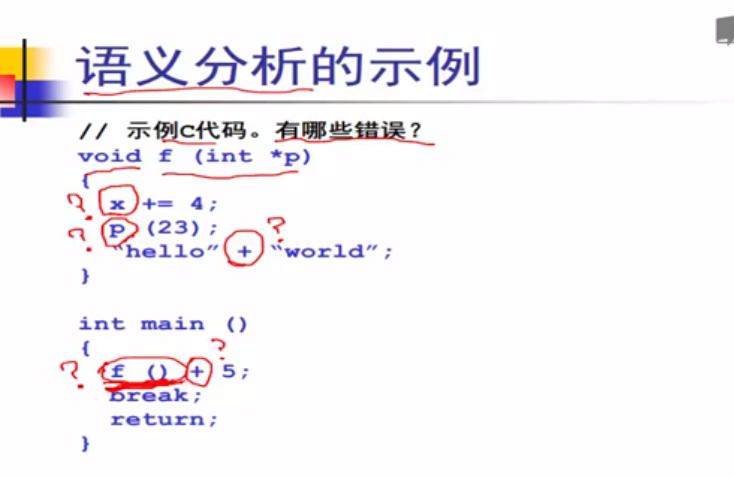语义分析依赖于上下文分析

抽象语法树一定是符合语法的，不会存在语法错误，语义分析器会分析语义是否满足。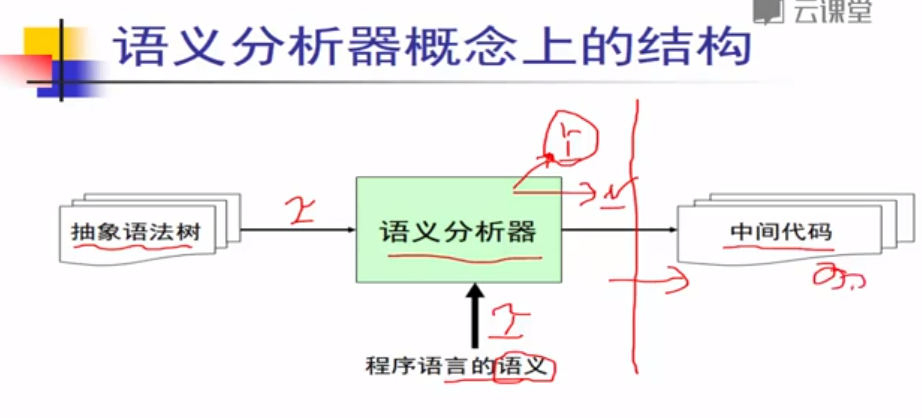如果中间代码之后，仍然有问题，只可能是编译器的问题。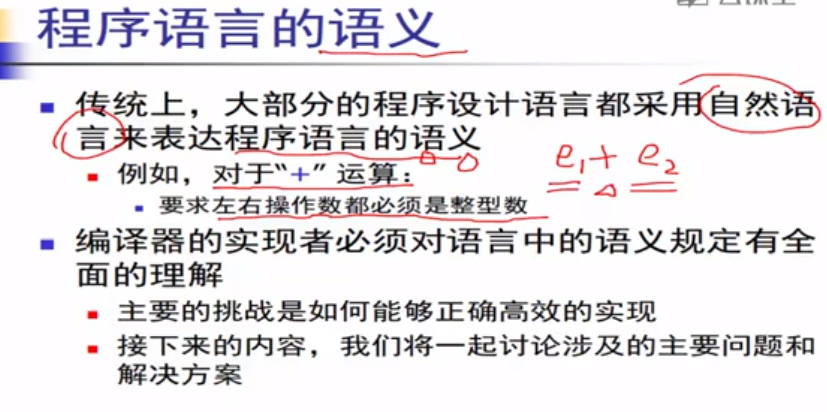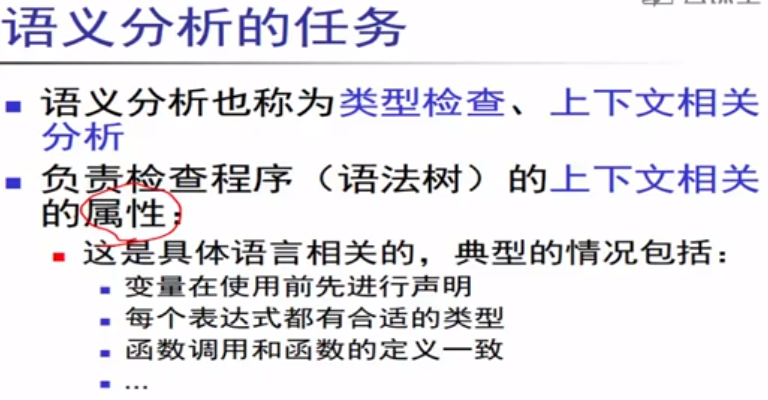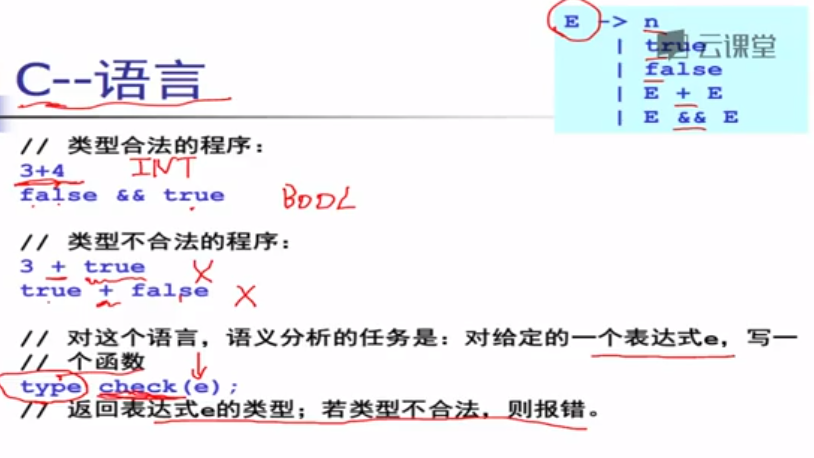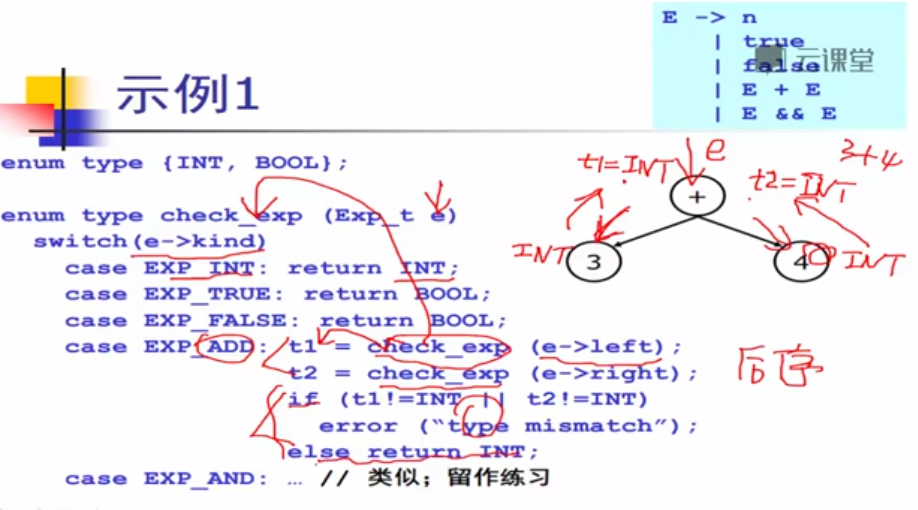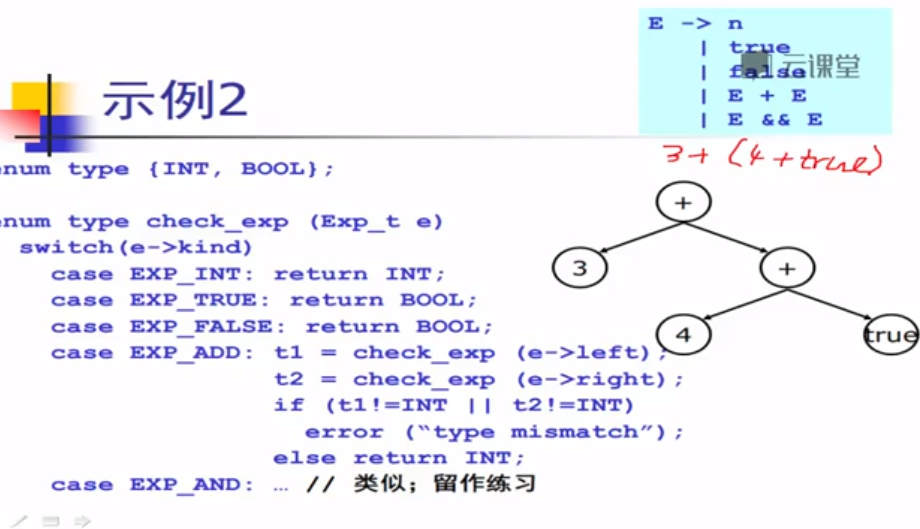D 声明 declaration E 表达式expression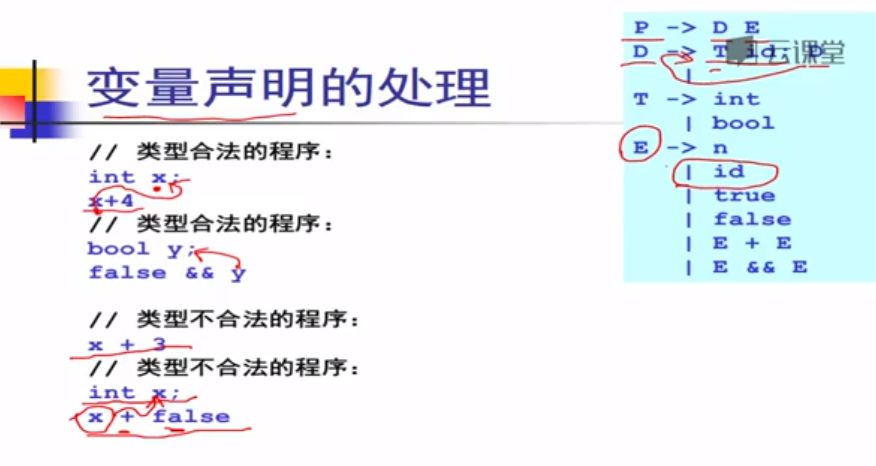涉及到变量声明的类型与使用时，进行计算的另一个元素类型一致。

检查变量声明（P，D）以及表达式（E）

符号表：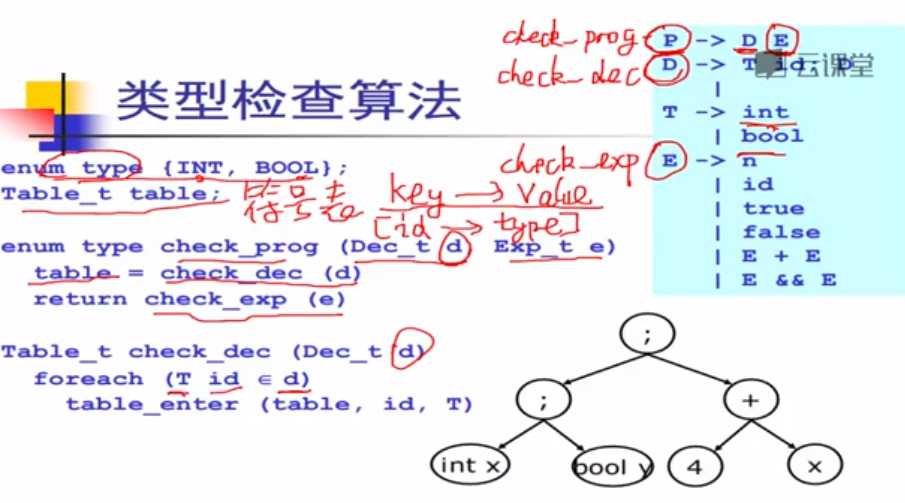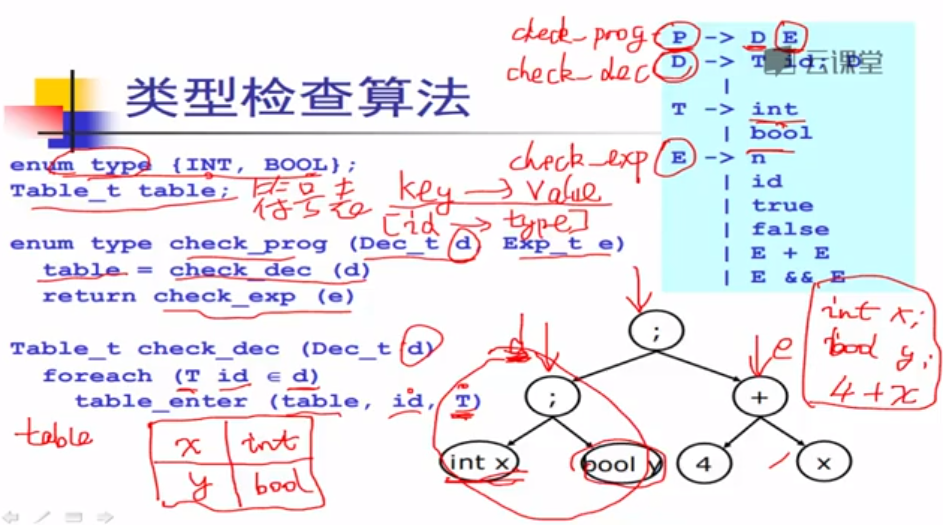处理声明语句时，得到符号表。处理表达式时，根据符号表查找，，返回相应的类型。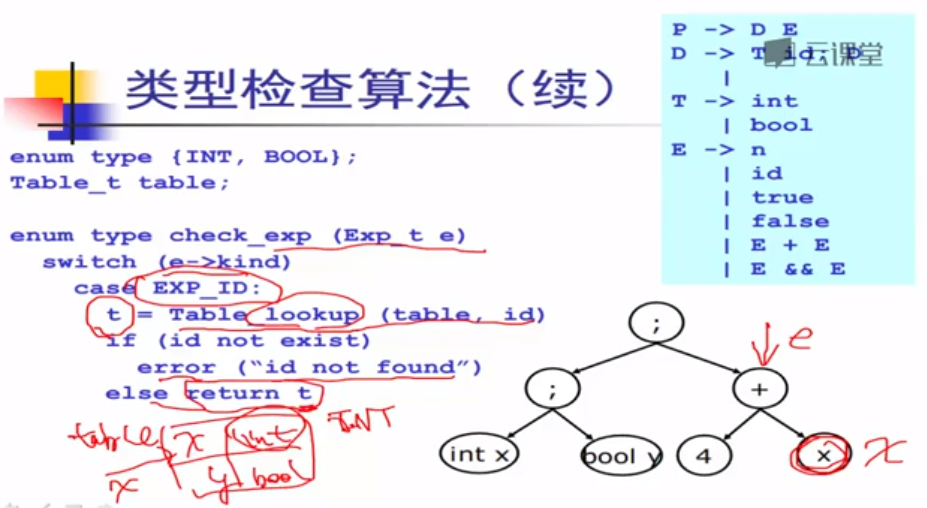后序遍历，先遍历负责声明左子树，生成符号表，根据符号表，再递归右边时，可以根据符号表进行表达式的类型检查。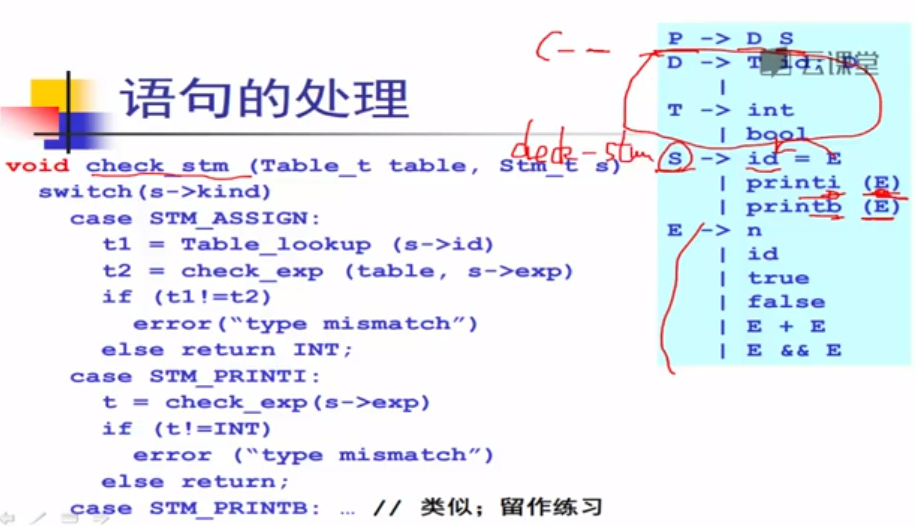符号表：存储变量相关的信息，后续也可以使用。

、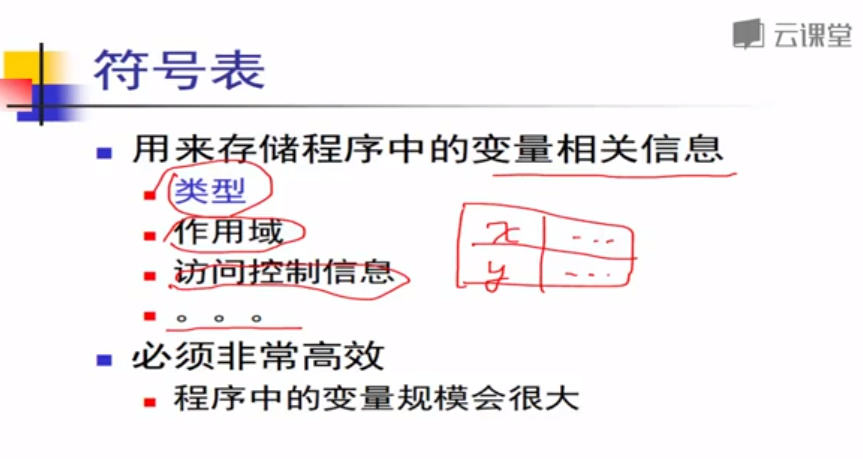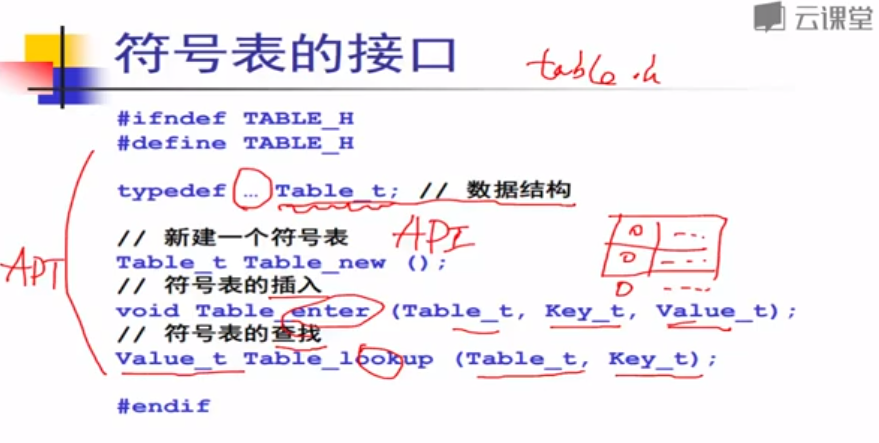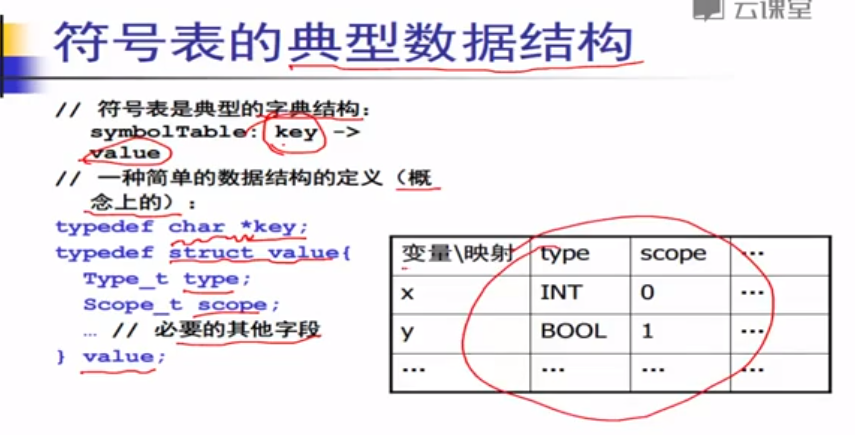哈希表速度快，但是会因为装在因子的存在，而存在浪费空间的问题；

如果改为红黑树，则节省空间。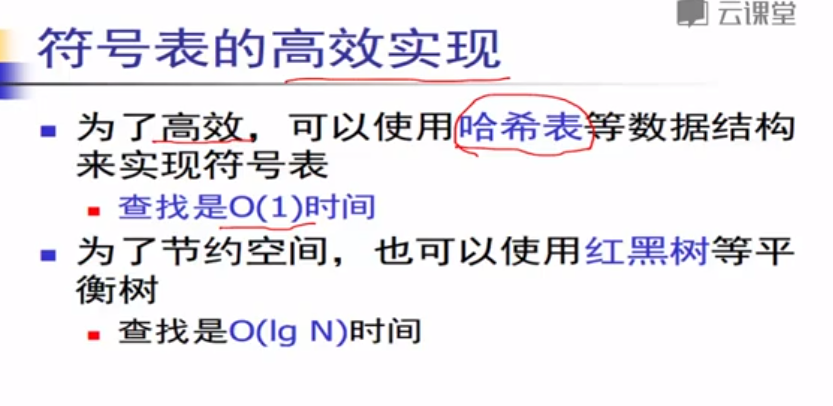作用域：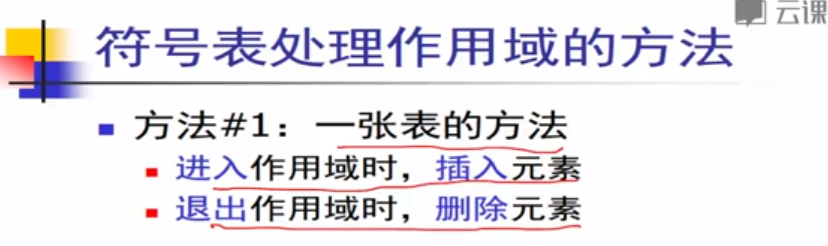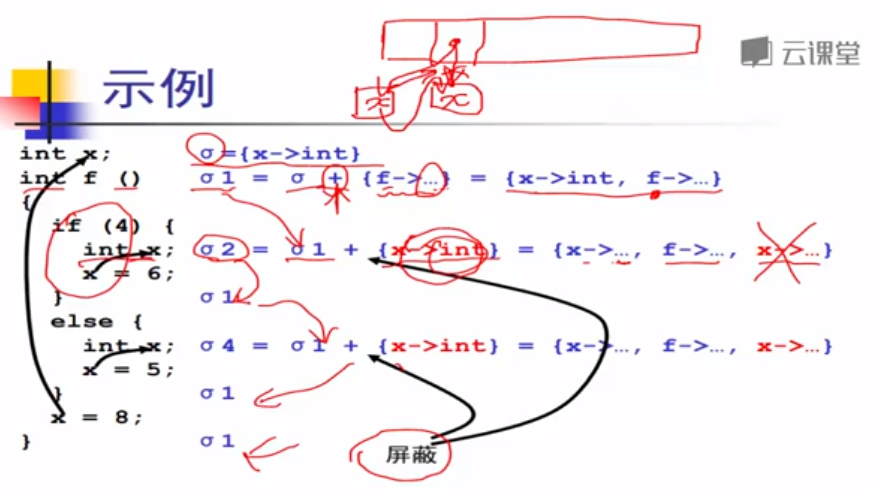方法2.做一个符号表的栈，每个元素是一个符号表，栈顶是最里层的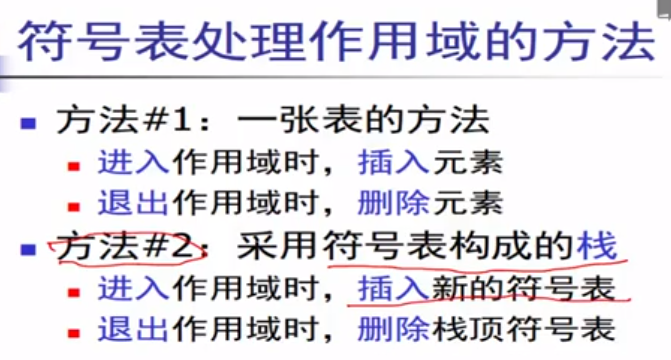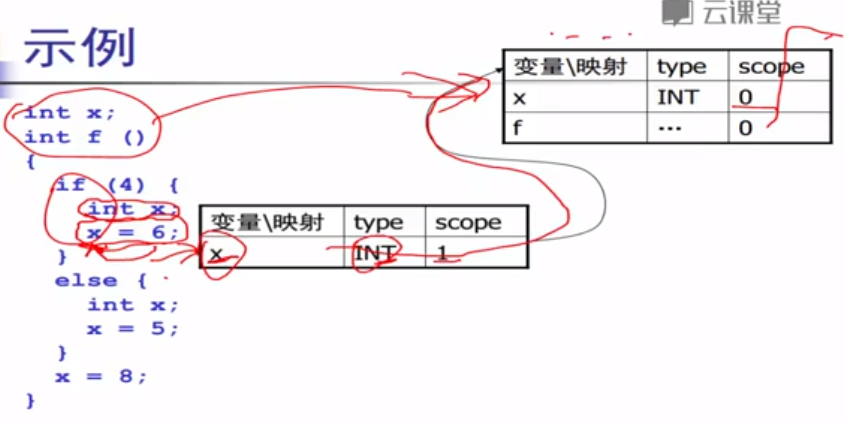符号表的一个比较重要的作用：名字空间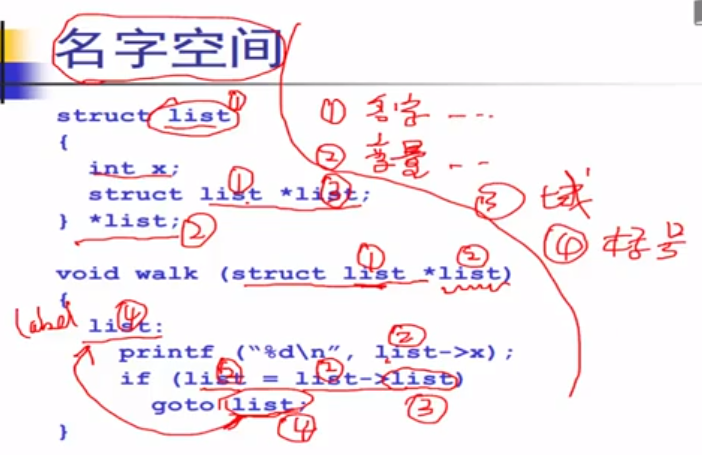不同的空间有不同的符号表，因此即使不同的符号表中有相同的名字，也是没有问题的。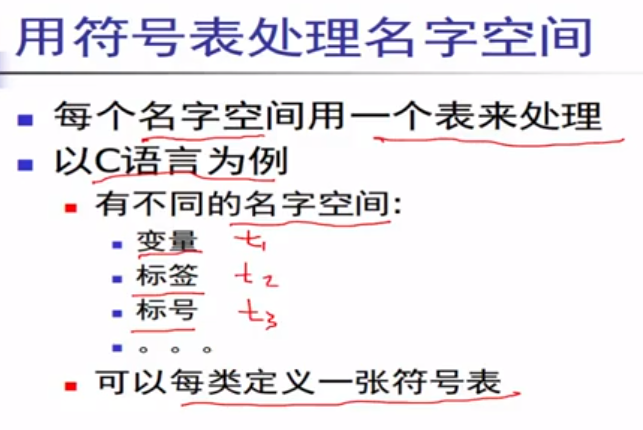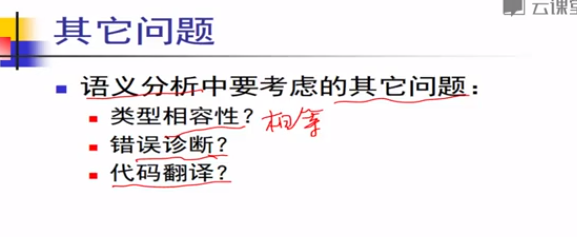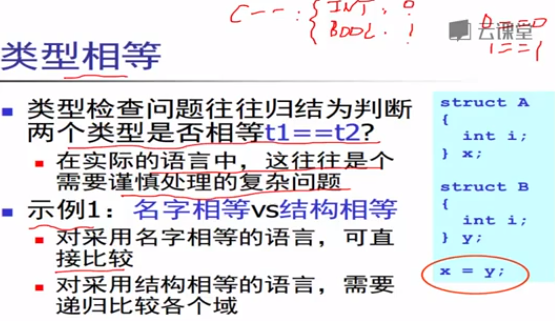虽然类型不同，但是因为是子类赋值给父类，因此是可以的，因此在符号表中需要维护继承关系。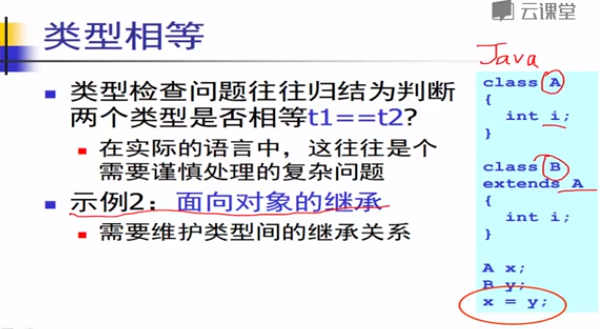位置信息需要从词法到语法到语义完整的保留，直到语义，因为语义之后信息都是争取的。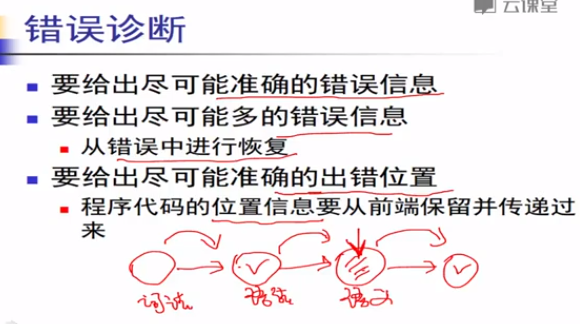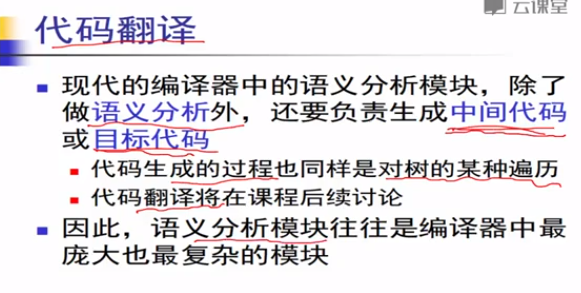展开全文• //调用expression(其实就是获取完下一个数据单位后，在factor函数中对其分析,当下一个数据单位不为(,获取下一个数据单位，且执行下一句)  if(syn==28)//如果下一个数据单位为')'  scaner();//直接获取下一个...
#include<stdio.h>
#include<string.h>
#include<conio.h>
#include<malloc.h>
#include<STDLIB.H>
{
char result;
char ag1;
char op;
char ag2;
};
int count=0;
char *expression(void);
char prog,token;//function长度为8，加上'\0'，所以数组最小大小应该为9
char ch;
int syn,p,m,n,sum=0;
int kk=0,k=0;
char *rwtab={"function","if","then","while","do","endfunc"};
void scaner()
{
m=0; ch=prog[p++];//ch为读入第一个数据（一个新的字符串中第一个数据，并不代表整个文本第一个数据）
for(n=0;n<8;n++)//初始化token数组
token[n]='\0';
while(ch==' ')
ch=prog[p++]; //跳过空格
if((ch>='a' && ch<='z')||(ch>='A' && ch<='Z'))//如果读入的第一个数据为字符 (字符串先存放在token数组中) {
while((ch>='a' && ch<='z')||(ch>='A' && ch<='Z')||(ch>='0'&&ch<='9'))  {
token[m++]=ch;//暂时存放在token数组中
ch=prog[p++]; }//字符串
token[m++]='\0';
p--;//使得prog[p]被ch读入的最后一个字符
syn=10;//用来区分输入数据类型
for(n=0;n<6;n++) {
if(strcmp(token,rwtab[n])==0)//判断读入字符串是否为程序中定义的特殊字符串{
syn=n+1;//function:1,if:2,then:3,while:4,do:5,endfunc:6break;} } }
else if(ch>='0' && ch<='9')//读入第一个数据为数字(读入为数字，直接经过sum运算，结果存放在sum中) {
sum=0;
while(ch>='0' && ch<='9') {
sum=sum*10+ch-'0';//将输入数字转换成10进制数
ch=prog[p++];}
p--;//使得prog[p]被ch读入的最后一个数字
syn=11;//若为数字，则,syn为11 }
else switch(ch)//读入第一个数据为运算符(运算符先存放在token数组中) {
case '<':         m=0;token[m++]=ch;//token='<',m=1
ch=prog[++p];//ch取下一个数据
if(ch=='=')//<= {
syn=22;  //token[m+1]=ch;这一句的意思是token=ch,错误，修改如下 token[m++]=ch;//token='=' }
else//< {
syn=20;  ch=prog[--p];//ch='<' }
break;
case '>':         m=0;token[m++]=ch;
ch=prog[++p];
if(ch=='=')  {
syn=24; token[m++]=ch; }
else  {
syn=23; ch=prog[--p];  }
break;
case '=':     m=0;token[m++]=ch;
ch=prog[++p];
if(ch=='=') {
syn=25; token[m++]=ch; }
else {
syn=18; ch=prog[--p]; }
break;
case '!':     m=0;token[m++]=ch;
ch=prog[++p];
if(ch=='=') {
syn=22; token[m++]=ch;  }
else syn=-1;
break;
case '+':syn=13;token=ch;break;
case '-':syn=14;token=ch;break;
case '*':syn=15;token=ch;break;
case '/':syn=16;token=ch;break;
case ';':syn=26;token=ch;break;
case '(':syn=27;token=ch;break;
case ')':syn=28;token=ch;break;
case '#':syn=0;token=ch;break;
default:syn=-1; }
}
/*该函数的功能是生成一个三地址语句送到四元式表中。*/
void emit(char *result,char *ag1,char *op,char *ag2)
{
count++;
return;
}
/*该函数回送一个新的临时变量名，临时变量名产生的顺序为t1，t2，…*/
char *newtemp()
{  //k初值为0
char *p; char m;
p=(char *)malloc(8);
k++;//假设第一次调用该函数，此时k=1;
itoa(k,m,10);//m=1;
strcpy(p+1,m);//把m的值复制到p指针指向的地址后面一个字节开始的内存中,p=1
p='t';
return(p);
}
/*factor函数，检查是否标识符，如果是，调用scaner函数，
如果不是，检查是否是数值，
如果是，调用scaner函数，
如果不是，检查是否是'('，
如果不是，进行出错处理，
如果是，调用scaner函数，再调用expression()函数，返回后检查是否是')',
如果不是，进行出错处理，如果是，调用scaner函数，返回*/
char *factor(void)
{
char *fplace;
fplace=(char *)malloc(12);
strcpy(fplace," ");//初始化fplace
if(syn==10)//该数据单位为字符串
{
strcpy(fplace,token);//将该字符串赋值给fplace
scaner();//获取下一个数据单位
}
else if(syn==11)//该数据单位为数字
{
itoa(sum,fplace,10);//将sum的值以10进制的形式存放在fplace中
scaner();//获取下一个数据单位
}
else if(syn==27)//该数据单位为({
scaner();//获取下一个数据单位
fplace=expression();//调用expression(其实就是获取完下一个数据单位后，在factor函数中对其分析,当下一个数据单位不为(,获取下一个数据单位，且执行下一句)
if(syn==28)//如果下一个数据单位为')'
scaner();//直接获取下一个数据单位
else   {
printf("\n')'错误");
kk=1; //标志  } }
else{
printf("\n')'错误");
kk=1; }
return (fplace);//返回数据单位的内容，如果是(,则返回(后面一个数据单位的内容
}
/*term()函数的作用是判断输入的是否是由'*''/'连接成的因式,并将对应的四元式保存并输出*/
char *term(void)
{
char *tp,*ep2,*eplace,*tt;
tp=(char *)malloc(12);
ep2=(char *)malloc(12);
eplace=(char *)malloc(12);
tt=(char *)malloc(12);
strcpy(eplace,factor());//调用factor,eplace为上一个数据单位的内容
while(syn==15||syn==16)//该数据单位为'*'或者'/'{
if(syn==15)//如果为'*' {
tt='*'; tt='\0';//tt存放该数据单位的内容
}
else if(syn==16)//如果为'/'{
tt='/'; tt='\0';//tt存放该数据单位的内容}
scaner();//获取下一个数据单位
strcpy(ep2,factor());//调用factor()函数，并将函数返回值赋值给ep2(字符串，数字，'(',出错)
strcpy(tp,newtemp());//调用newtemp()函数，将该函数返回值赋值给tp,tp为临时变量名
emit(tp,eplace,tt,ep2);//调用emit函数,生成一个三地址语句存放在四元式表中
strcpy(eplace,tp);//eplace为四元式}
return(eplace);
}
char *expression(void)
{
char *tp,*ep2,*eplace,*tt;
tp=(char *)malloc(12);
ep2=(char *)malloc(12);
eplace=(char *)malloc(12);
tt=(char *)malloc(12);
strcpy(eplace,term());//调用term函数,将四元式的内容赋值给eplace
while(syn ==13 || syn==14) //当它不为*或者/时，接着判断是否为+或者-
{
if(syn==13)
{
tt='+';
tt='\0';
}
else if(syn==14)
{
tt='-'; tt='\0';
}
scaner();
strcpy(ep2,term());
strcpy(tp,newtemp());
emit(tp,eplace,tt,ep2);
strcpy(eplace,tp);
}
return(eplace);//返回对应四元式的值
}
int statement()
{
char tt,eplace;
int schain=0;
switch(syn)
{
case 10: strcpy(tt,token);//若第二个数据单位的syn为10，即字符串(as1),将第二个数据单位的内容复制给tt
scaner();//再取下一个数据单位，并获得该数据单位的syn
if(syn==18)//第三个数据单位为'='{
scaner();//再取下一个数据单位，并获得该数据单位的syn(第四个数据单位)
strcpy(eplace,expression());//调用expression,如果为+或者-,返回对应四元式的值，如果为*或者/,则返回其四元式的值
emit(tt,eplace," "," ");
schain=0;
}
else {
printf("\n缺少赋值句\n"); kk=1; }
break;   }
return(schain);
}
int yucu()
{
int schain=0;
schain=statement();//调用statement()函数，
while(syn==26)//该数据单位为; {
scaner();
schain=statement();
}
return(schain);
}
int lrparser()
{
int schain=0; kk=0;
if(syn==1)//输入数据为function {
scaner();//因为p停留在上一次扫描结束的地方，所以再次调用该函数ch可以获取下一个数据单位，得到该数据单位的syn
schain=yucu();//调用yucu()函数
if(syn==6)//endfunc {
scaner();
if(syn==0 && kk==0)//#
printf("\n语法，语义分析成功!");}
else {
if(kk!=1) {
printf("\n缺endfunc\n"); kk=1;} } }
else  {
printf("\n缺少function\n");
kk=1; }
return(schain);
}
void main()
{ int i; p=0; FILE *fp;//if((fp=fopen("D:\\6.txt","r"))==NULL)if((fp=fopen("E:\\编译原理期末代码\\36.txt","r"))==NULL){
printf("\n 文件打开失败:\n");   exit(0);}do{ ch=fgetc(fp);
prog[p++]=ch;
}while(ch!='#'); //将输入字符串赋值到prog数组
p=0; //使得ch从第一个字符开始获取
printf("种别码 单词符号\n");
do{
scaner(); //扫描输入的数据，并作出类型判断(以字符串为单位)
switch(syn) {
case 11: printf("%-3d %d\n",syn,sum); break; //输入为数字,打印出它的syn与大小
case -1: printf("词法分析失败,程序终止!\n"); return; //输入数据不正确
default: printf("%-3d %s\n",syn,token); //字符串，运算符} }while(syn!=0);//读入所有输入的数据(只是判断输入数据是否正确,并没有进行语法，语义分析操作)
printf("词法分析成功，按任意键进行语法、语义分析");
getch();//按任意键，程序往下执行
p=0;//词法分析后，重新对ch赋值，使得ch从第一个字符开始获取
scaner();//扫描第一个单位，判断其类型
lrparser();//将将第一个输入字符串翻译为四元式
if(kk!=0)
{
printf("语法分析失败，程序终止!");
return;
}
printf("\n三地址指令如下:\n");
for(i=0;i<count;i++)
{
}
getch();
return;
}


展开全文• 附录c 编译程序实验 实验目的：用c语言对一个简单语言的子集编制一个一遍扫描的编译程序，以加深对编译原理的理解,掌握编译程序的实现方法和技术。 语法分析 C2.1 实验目的 编制一个递归下降分析程序,实现对词法分析...
• 云南大学编译原理实验期中作业，语义分析编译通过可直接运行内含测试文件
• 2. 理解语法、语义分析程序为中心的单遍编译程序组织方法； 3. 理解语义分析的基本机制； 4. 掌握语义子程序的构造方法； 5. 理解编译程序的基本逻辑过程（词法分析、语法分析、语义分析及目标代码的生成； 6. ...
云南大学编译技术实验报告

一、实验目的
1.  进一步理解递归下降分析原理和实现方法；
2.  理解语法、语义分析程序为中心的单遍编译程序组织方法；
3.  理解语义分析的基本机制；
4.  掌握语义子程序的构造方法；
5.  理解编译程序的基本逻辑过程（词法分析、语法分析、语义分析及目标代码的生成；
6.  理解编译过程中的符号表的基本管理方法；

二、实验内容
文法G[E]如下：
E→E+T | E-T |T
T→T*F | T/F | F
F→( E )
F→ id
注 id 为字母打头后面是数字或者字母的串；
在starter files基础上
1.  实现增加乘法 * 和除法 / 运算，并且，/优先级别高于+；其次，和/满足左结合规则；
2.  对包含乘除法运算的表达式生成对应的四元式；
3.  禁止同名重复声明，所以登记符号之前要检查有没有同名变量声明过；
4.  增加类型检查；

三、实验的分析与设计
1.经过修改后的翻译模式：
start->   DS.
D->B; D
D->ε
B->int L    { L.type := int }|real L    { L.type := real }
L->id   { A.Type := L.type  enter(v.entry,L.type)}  A
A-> ，idA   { A1.Type := A.type  enter(v.entry,A.type)}
A->ε
S→ V := E { gen( “:=”, E.place,0,V.place) } H
H→；S | ε
E->T    { R.i:=T.place}      R     {E.place:=R.s}
R->+T    { R1.i:= newtemp; gen( “*”, R.i, T.place , R1.i) }     R   {R.s:= R1.s; }
R-> ε    {Rs=R.i}

T→F  {p.i:=F.place}   P   {T.place:=P.s}
P->F  {p1.i:=newtemp;gen(““,P.i,T.place,P.i)}  P  {P.s:=P1.s;}
P->/F  {p1.i:=newtemp;gen(“/”,P.i,T.place,P.i)}  P  {P.s:=P1.s;}
P-> ε    {Ps=P.i}

F->( E )  { F.place := E.place}
F->id     {F.place:=position (id)}
V->id     {V.place:=position(id)}

type、i属性为继承属性，place、s属性为综合属性。

实现增加乘法 * 和除法 / 运算，并且，/优先级别高于+；其次，和/满足左结合规则；
对包含乘除法运算的表达式生成对应的四元式；
E->T    { R.i:=T.place}      R     {E.place:=R.s}
R->+T    { R1.i:= newtemp; gen( “*”, R.i, T.place , R1.i) }     R   {R.s:= R1.s; }
R-> ε    {Rs=R.i}
T→F  {p.i:=F.place}   P   {T.place:=P.s}
P->F  {p1.i:=newtemp;gen(““,P.i,T.place,P.i)}  P  {P.s:=P1.s;}
P->/F  {p1.i:=newtemp;gen(“/”,P.i,T.place,P.i)}  P  {P.s:=P1.s;}
P-> ε    {Ps=P.i}
3.  禁止同名重复声明，所以登记符号之前要检查有没有同名变量声明过；
登记符号之前要检查有没有同名变量声明过。
在enter( )这个存放声明变量的函数中，增加相应检验变量名重复的函数：

增加类型检查；
检验’:=’前后字符类型是否匹配
检验’+’前后类型是否匹配

检验’*’前后的类型是否匹配

检验’/’前后的类型是否匹配

四、实验的实现

五、运行的结果以及存在问题及其原因

#include <stdio.h>
#include<dos.h>
#include<stdlib.h>
#include<string.h>
//#include <iostream.h>
#include<ctype.h>
#include <windows.h>

#define txmax 100    /* 符号表最大容量 */
#define al 10        /* 符号的最大长度 */
#define tvmax 500    /* 最多能够申请的临时变量取值范围[100, tvmax] */
#define norw 2       /* 关键字个数 */
#define cxmax 500   /* 最多的虚拟机代码数 */

int tx;              //名字表指针, 取值范围[0, txmax-1]
int tv ;             //临时变量计数

/* 符号 */
enum symbol {
nul,         eeof,  ident,         plus,      times,    lparen,divide,
rparen,    comma,     semicolon,  becomes,  period, realsym,    intsym,
};

enum symbol sym;    /* 当前的符号 */
char ch;            /* 获取字符的缓冲区，getch 使用 */
char id[al+1];      /* 当前ident, 多出的一个字节用于存放0 */
char a[al+1];       /* 临时符号, 多出的一个字节用于存放0 */

/* 符号表结构 */
struct tablestruct
{
char name[al];      /* 名字 */
enum symbol type;   // 类型
};

struct tablestruct table[txmax]; /* 符号表 */

char word[norw][al];        /* 保留字 */
enum symbol wsym[norw];     /* 保留字对应的符号值 */
enum symbol ssym;      /* 单字符的符号值，散列表 */

int cx;             /* 四元式代码指针, 取值范围[0, cxmax-1]*/
struct instruction
{
char f[al+1]; /* 操作码 */
char l[al+1];     /* 左操作数 */
char r[al+1];     /* 右操作数*/
char t[al+1];     /* 结果 */
};
struct instruction code[cxmax]; /* 存放虚拟机代码的数组 */

FILE* fin;
FILE* fout;

int getsym();
void enter(enum symbol type);
void init();
int position(char* idt);
int gen(enum symbol op, int arg1, int arg2,int result );     //中间代码分析
void writecode(char *op, char *arg1, char *arg2,char *result );    //写缓存
void  start();
void  D();
void  B();
void L(enum symbol type);
void A(enum symbol type);
void S();
void H();
int E();
int R(int Ri);
int T();
int P(int Pi);
int F();
int V();


#include "语义分析.h"

int  main()
{
char filename;

printf("请输入分析的文件名:");
gets(filename);
if((fin=fopen(filename,"r"))==NULL)
{
printf("不能打开文件.\n");
exit(0);
}

init();                  //初始化
getsym();                //读第一个单词，将单词类别放入sym中，单词值放入id中
start();                //开始按start->DS.  分析

if (sym==eeof)
{
printf("语法正确\n\n将中间代码保存到文件请输入文件名，否则回车");
gets(filename);
if(strlen(filename)<=0) return 0;
if((fout=fopen(filename,"w"))==NULL)
{
printf("不能打开文件.\n");
exit(0);
}
for (int cx1=0; cx1<cx; cx1++)
fprintf(fout,"(%s,%s,%s,%s)\n",code[cx1].f,code[cx1].l,code[cx1].r,code[cx1].t);
return 0;
}
else
{
printf("语法错1:  . 后不能再有句子");
exit(0);
}
fclose(fin);
fclose(fout);
return 0;
}

//初始化函数
void init()
{
int i;

/* 设置单字符符号 */
for (i=0; i<=255; i++)
{
ssym[i] = nul;            //不正确的单字符符号为nul，先预置初值nul
}
ssym['+'] = plus;
ssym['*'] = times;
ssym['('] = lparen;
ssym[')'] = rparen;
ssym['.'] = period;
ssym[','] = comma;
ssym[';'] = semicolon;
ssym['/']=divide;

/* 设置保留字名字 */
strcpy(&(word), "real");
strcpy(&(word), "int");

/* 设置保留字符号 */
wsym = realsym;
wsym = intsym;

tv=100;           //临时变量指针初值，让Tx和tv的取值没有交集，区别到底是临时变变量和声明的变量
tx=0;           //表指针初值
cx=0;     //指令计数器

}

/*
* 词法分析，获取一个符号
*/
int getsym()
{
int i,k;
ch=fgetc(fin);

if (ch==EOF)
{
sym=eeof;    //文件结束
return 0;
}

while (ch==' ' || ch==10 || ch==13 || ch==9)  /* 忽略空格、换行、回车和TAB */
ch=fgetc(fin);

if (ch>='a' && ch<='z')
{
/* 名字或保留字以a..z开头 ，*/
k = 0;
do
{
if(k<al)                  /* 符号的最大长度为al ，超长就截尾处理*/
{
a[k] = ch;
k++;
}
ch=fgetc(fin);
}
while ((ch>='a' && ch<='z' )|| (ch>='0' && ch<='9'));

a[k] = 0;
strcpy(id, a);//a的地址赋予id
fseek(fin,-1,1);//文件指针偏移，向后读一个

for (i=0; i<norw; i++)      /* 搜索当前符号是否为保留字 */
if (strcmp(id,word[i]) == 0)//如果是
break;
if (i <norw)//已经经过定义，不可作为变量和过程名
{
sym = wsym[i];
}
else
{
sym = ident;          /* 搜索失败则，类型是标识符 */
}
}
else if(ch == ':') /* 检测赋值符号 */
{
ch=fgetc(fin);
if (ch == '=')
{
sym = becomes;
}
else
{
sym = nul;  /* 不能识别的符号 */
}
}
else sym = ssym[ch];     /* 当符号不满足上述条件时，全部按照单字符符号处理 */
return 0;
}

/*
* 在符号表中加入一项
*/

void enter(enum symbol type)//将声明过的变量保存在结构体table[tx]中
{
tx=tx+1;
if (tx > txmax)
{
printf(" 符号表越界 ");           /* 符号表越界 */

return;
}
for(int i=1; i<txmax; i++)
{
if(strcmp(id,table[i].name)==0)
{
printf("错误1：变量名重复定义\n");
exit(0);
}
}
strcpy(table[tx].name, id); /* 全局变量id中已存有当前名字的名字,Tx为插入当前符号之前表尾指针 */
table[tx].type = type;

}

/*
* 查找名字的位置.
* 找到则返回在名字表中的位置,否则返回0.
*
* idt:    要查找的名字
* tx:     当前名字表尾指针，全局变量
*/
/*判断是否已经存在*/
int position(char* idt)
{
int i;
strcpy(table.name, idt);
i = tx;
while (strcmp(table[i].name, idt) != 0)
{
i--;
}
return i;
}

/* 中间代码，生成四元式，目标代码*/
int gen(enum symbol op, int arg1, int arg2,int result )//通过传来的参数产生四元式
{
char temp1[al+1],temp2[al+1],temp3[al+1];
if(arg1>=100)                            //模拟申请临时变量
{
wsprintf(temp1,"T%d",arg1);//temp1是一个缓冲区，将arg1内容输出到temp1中
}
else
{
strcpy(temp1, table[arg1].name);//把table[arg1].name的内容copy到temp1中
}

if(arg2>=100)
{
wsprintf(temp2,"T%d",arg2);
}
else
{
strcpy(temp2, table[arg2].name);
}

if(result>=100)
{
wsprintf(temp3,"T%d",result);
}
else
{
strcpy(temp3,table[result].name);
}

if (op==becomes)//赋值符
{
if(table[arg1].type!=table[result].type) //检验':='前后字符类型是否匹配
{
printf("(:=,%s,%s,%s)\n",temp1,temp2,temp3);//输出四元式
writecode(":=",temp1,temp2,temp3);//写入缓存
printf("语法错：%s和%s的类型不一致\n",temp1,temp3);//':='前后字符类型不匹配，报错

}
else //:=前后字符类型匹配
{
printf("(:=,%s,%s,%s)\n",temp1,temp2,temp3);
writecode(":=",temp1,temp2,temp3);//写入缓存

}
}

else if (op==plus)                          //+运算
{

if(table[arg1].type==table[arg2].type)//检验'+'前后类型是否匹配
{
table[result].type=table[arg1].type;
writecode("+",temp1,temp2,temp3);
printf("(+,%s,%s,%s)\n",temp1,temp2,temp3);
}

else
{
printf("(+,%s,%s,%s)\n",temp1,temp2,temp3);
writecode("+",temp1,temp2,temp3);
printf("语法错：%s和%s的类型不一致\n",temp1,temp2);
}
}

/*乘法*/
else if(op==times)
{
if(table[arg1].type==table[arg2].type)//检验'*'前后的类型是否匹配
{
table[result].type=table[arg1].type;
printf("(*,%s,%s,%s)\n",temp1,temp2,temp3);
writecode("*",temp1,temp2,temp3);
}
else
{
printf("(*,%s,%s,%s)\n",temp1,temp2,temp3);
writecode("*",temp1,temp2,temp3);
printf("语法错：%s和%s的类型不一致\n",temp1,temp2);

}

}

/*除法*/
else if(op==divide)
{
if(table[arg1].type==table[arg2].type)//检验'/'前后的类型是否匹配
{
table[result].type=table[arg1].type;
printf("(/,%s,%s,%s)\n",temp1,temp2,temp3);
writecode("/",temp1,temp2,temp3);
}
else
{
printf("(/,%s,%s,%s)\n",temp1,temp2,temp3);
writecode("/",temp1,temp2,temp3);
printf("语法错：%s和%s的类型不一致\n",temp1,temp2);

}
}
return 0;
}

//处理代码段，打印
void writecode(char op[al+1], char arg1[al+1], char arg2[al+1],char result[al+1] )
{
if (cx >= cxmax)
{
printf("Program too long"); /* 程序过长 */
return ;
}
strcpy(code[cx].f, op);
strcpy(code[cx].l,arg1);
strcpy(code[cx].r,arg2);
strcpy(code[cx].t,result);
cx++;
return ;
}

/*分析产生式     X->DS.   */
void  start()
{
if (sym==intsym||sym==realsym)//如果变量是int或者real型
{
D();
S();
if (sym==period)
{
getsym();//调用词法分析
return;
}
else
{
printf("语法错2： 缺少程序结束.");
exit(0);
}
}
else
{
printf("语法错3: 程序只能用int,和real开始，而且区分大小写");
exit(0);
}
}

/*递归下降分析
D->  B; D
D->ε

*/
void  D()
{
if (sym==intsym ||sym==realsym)
{
B();
if (ch=';')
{
getsym();
D();
}
else
{
printf("语法错4");
exit(0);
}
}
else if(sym==ident)  return;
else
{
printf("语法错5");
exit(0);
}
}

/*
B->   int L    { L.type := int }|real L    { L.type := real }
*/
void  B()
{
if (sym==intsym )//继承L的类型
{
getsym();
L(intsym);
}
else if (sym==realsym)
{
getsym();
L(realsym);
}
else
{
printf("语法错6：变量不是real或int型\n");
exit(0);
}
}

/*
L->   id   { A.Type := L.type  enter(v.entry,L.type)}   A       V.entry通过全局变量tx隐性传递
有参，有几个继承属性就有几个参数
*/
void L(enum symbol type)//L的id赋予A
{
int Vplace;
if (sym==ident)
{
Vplace=position(id);
if(Vplace>0)
{
printf("语法错7：变量重复声明\n");
exit(0);
}
enter(type);
getsym();
A(type);
}
else
{
printf("语法错8：缺少变量");
exit(0);
}
}

/*
A-> ，id  A   { A1.Type := A.type  enter(v.entry,A.type)}
A->ε
*/

void A(enum symbol type)
{
int Vplace;
if (sym==comma)          //当前单词为，
{
getsym();
if (sym==ident)
{
Vplace=position(id);
if(Vplace>0)
{
printf("语法错7：变量重复声明\n");
exit(0);
}
enter(type);
getsym();
A(type);
}
else
{
printf("语法错9");
exit(0);
}
}
else if (sym== semicolon)   return ;//当前单词为；即A的follow集元素相当于进行A->ε
else
{
printf("语法错10");
exit(0);
}
}

/*
S→ V := E { gen( ":=", E.place,0,V.place) } H
*/
void S()
{
int vplace,Eplace;
if (sym==ident)
{
vplace=V();
//getsym();
if (sym==becomes)     //当前单词为:=
{
getsym();
Eplace=E();
gen(becomes,Eplace,-1,vplace);
H();
}
else
{
printf("语法错11");
exit(0);
}
}
else
{
printf("语法错12");
exit(0);
}
}

/*
H→；S | ε
*/
void H()//执行下一条运算
{
if (sym==semicolon)          //当前单词为indent类型
{
getsym();
S();
}
else if (sym==period)   return ;
else
{
printf("语法错13");
exit(0);
}
}
/*
E->T      { R.i:=T.place}      R     {E.place:=R.s}
综合属性，有返回值
*/

int E()//分析赋值号后面的运算
{
int ri,tplace,Rs;
if (sym==ident || sym== lparen)//当前为标识符或左括号
{
tplace=T();
ri=tplace;
Rs=R(ri);
}
else
{
printf("语法错14");
exit(0);
}
return Rs;
}

/*
R->+T     { R1.i:= newtemp; gen( "*", R.i, T.place , R1.i) }     R   {R.s:= R1.s; }
R-> ε    {R.s=R.i}
R既有参数又有返回值
*/

int R(int Ri)//检验+运算
{
int Rs,tplace;
if (sym==plus)
{
getsym();
tplace=T();
tv=tv+1;            //生成临时变量
gen(plus,Ri,tplace,tv);
Rs=R(tv);
}
else if (sym== semicolon || sym==rparen|| sym==period)
{
Rs=Ri;
}
else
{
printf("语法错15");
exit(0);
}
return Rs;
}

/*实现乘除的左结合及优先级
F->( E )  { F.place := E.place}
F->id     {F.place:=position (id)}
*/
int F()//实现*、/运算的优先级高于+运算
{
int Fplace;
if(sym==ident)
{
Fplace=position(id);//获取当前位置
if(Fplace==0)
{
printf("变量没有声明\n");
exit(0);
}
getsym();
}
else if(sym==lparen)
{
getsym();
Fplace=E();
if(sym==rparen)getsym();
else
{
printf("语法错16，缺)");
exit(0);
}
}

else
{
printf("语法错17,，缺(");
exit(0);
}
return Fplace;
}

/*
P->*F  {p1.i:=newtemp;gen("*",P.i,T.place,P.i)}  P  {P.s:=P1.s;}
P->/F  {p1.i:=newtemp;gen("/",P.i,T.place,P.i)}  P  {P.s:=P1.s;}
P-> ε    {Ps=P.i}
*/
int P(int Pi)//检验*、/运算
{
int ps,Fplace;
if(sym==times)
{
getsym();
Fplace=F();
tv=tv+1;//生成临时变量
gen(times,Pi,Fplace,tv);
ps=P(tv);
}

else if(sym==divide)
{
getsym();
Fplace=F();
tv=tv+1;
gen(divide,Pi,Fplace,tv);
ps=P(tv);
}

else if(sym==plus||sym==semicolon||sym==rparen||sym==period)
//求出P的follow集，进行语法检查，如判断左括号是否匹配
{
ps=Pi;
}

else
{
printf("语法错18：缺少变量或(");
exit(0);
}

return ps;
}
/*
将T的位置指向新增加乘除
T→F  {p.i:=F.place}   P   {T.place:=P.s}
*/
int T()
{
int Pi,Fplace,Ps;
if(sym==ident||sym==lparen)
{
Fplace=F();
Pi=Fplace;
Ps=P(Pi);
}

else
{
printf("语法错19");
exit(0);
}
return Ps;
}

/*

V->id     {V.place:=position(id)}
*/
int V()
{
int Vplace;
if (sym==ident)
{
Vplace=position (id);
if (Vplace==0)
{
printf("变量没有声明");
exit(0);
}
getsym();
}
else
{
printf("语法错20");
exit(0);
}
return Vplace;
}

展开全文pl0编译器
• 编译原理实验报告：语义分析及中间代码生成1. 实验题目：语义分析及中间代码生成实验目的实验内容实验要求输入输出2. 设计思想3.算法流程4. 源程序5. 调试数据 1. 实验题目：语义分析及中间代码生成 实验目的 通过...
编译原理实验：语义分析及中间代码生成1. 实验题目：语义分析及中间代码生成实验目的实验内容实验要求输入输出2. 设计思想3.算法流程4. 源程序5. 调试数据
1. 实验题目：语义分析及中间代码生成
实验目的

通过上机实习，加深对语法制导翻译原理的理解，掌握将语法分析所识别的语法范畴变换为某种中间代码的语义翻译方法。
掌握目前普遍采用的语义分析方法──语法制导翻译技术。
给出PL/0文法规范，要求在语法分析程序中添加语义处理，对于语法正确的表达式，输出其中间代码；对于语法正确的算术表达式，输出其计算值。

实验内容
已给PL/0语言文法，在实验二或实验三的表达式语法分析程序里，添加语义处理部分，输出表达式的中间代码，用四元式序列表示。
实验要求

语义分析对象重点考虑经过语法分析后已是正确的语法范畴，本实验重点是语义子程序。
在实验二或实验三“语法分析器”的里面添加PL/0语言“表达式”部分的语义处理，输出表达式的中间代码，计算表达式的语义值。
中间代码用四元式序列表示。

输入输出

PL/0算术表达式的语义计算：
输入：
PL/0算术表达式，例如: 2 + 3 * 5作为输入。
输出：
17
PL/0表达式的中间代码表示
输入：
PL/0表达式，例如: a *（b +c）
输出：
( + b  c  t1 )
( * a  t1  t2 )

2. 设计思想
本次实验我采用的递归下降分析器的设计。
递归下降分析法的原理是利用函数之间的递归调用来模拟语法树自上而下的构建过程。从根节点出发，自顶向下为输入串中寻找一个最左匹配序列，建立一棵语法树。在不含左递归和每个非终结符的所有候选终结首字符集都两两不相交条件下，我们就可能构造出一个不带回溯的自顶向下的分析程序，这个分析程序是由一组递归过程（或函数）组成的，每个过程（或函数）对应文法的而一个非终结符。
语法：
<表达式> -> [+|-]<项>{<加法运算符> <项>}
<项> -><因子>{<乘法运算符> <因子>}
<因子> -> <标识符>|<无符号整数>|(<表达式>)
<加法运算符> -> +|-
<乘法运算符> -> *|/
计算FIRST集：
FIRST(<表达式>)={ +， -， (， <标识符>， <无符号整数> }
FIRST(<因子>)={ <标识符>， <无符号整数>， ( }
FIRST(<项>)={ <标识符>， <无符号整数>， ( }
FIRST(<加法运算符>)={ +， - }
FIRST(<乘法运算符>)={ *， / }
计算FOLLOW集：
FOLLOW(<表达式>)={ ) }
FOLLOW (<加法运算符>)={ <标识符>， <无符号整数>， ( }
FOLLOW (<乘法运算符>)={ <标识符>， <无符号整数>， ( }
可以改成如下拓广文法：
<表达式>-><项> | <表达式>+<项> | <表达式>-<项>
<项>-><因子> | <项>*<因子> | <项>/<因子>
<因子>->(<表达式>) | <标识符> | <无符号整数>
将语法消除左递归可得(用E代表<表达式>，T代表<项>，F代表<因子>)
E->TE’
E’->ɛ|+TE’|-TE’
T->FT’
T’->ɛ|*FT’|/FT’
F->ident|(E)|number
属性文法：是在上下文无关文法的基础上为每个文法符号（终结符或非终结符）配备若干个相关的“值”（称为属性）。
属性:代表与文法符号相关的信息，和变量一样，可以进行计算和传递。
综合属性
用于“自下而上”传递信息
在语法树中，一个结点的综合属性的值，由其子结点的属性值确定
S—属性文法：仅仅使用综合属性的属性文法
语义规则： 属性计算的过程即是语义处理的过程
对于文法的每一个产生式配备一组属性的计算规则，则称为语义规则。
(1)终结符只有综合属性，它由词法分析器提供
(2)非终结符既可以有综合属性也可以有继承属性，文法开始符号的所有继承属性作为属性计算前的初始值。
(3) 产生式右边符号的继承属性和产生式左边符号的综合属性都必须提供一个计算规则
(4) 产生式左边符号的继承属性和产生式右边符号的综合属性不由所给的产生式的属性计算规则进行计算，它们由其它产生式的属性规则计算
一遍扫描的处理方法： 与树遍历的属性计算方法不同，一遍扫描的处理方法是在语法分析的同时计算属性值，而不是语法分析构造语法树之后进行属性的计算，而且无需构造实际的语法树。
因为一遍扫描的处理方法与语法分析器的相互作用，它与下面两个因素密切相关：
1.所采用的语法分析方法
2.属性的计算次序
如下为递归下降分析器的设计实现思想

对每个非终结符A构造一个函数过程，对A的每个继承属性设置一个形式参数，函数的返回值为A的综合属性（作为记录，或指向记录的一个指针，记录中有若干域，每个属性对应一个域）。
非终结符A	对应的函数过程中，根据当前的输入符号决定哪个产生式候选。
每个产生式对应的代码中，按照从左到右的次序，对于单词符号（终结符），非终结符和语义分析分别做以下的工作。
（1）对于带有综合属性x的终结符X，把x的值存入为X.x设置的变量中。然后产生一个匹配X的调用，并继续读入一个输入符号。
（2）对于每个非终结符号B，产生一个右边带有函数调用的赋值语句c=B(b1,b2,…，bk)
（3）对于语义动作，把动作的代码抄进分析器中，用代表属性的变量来代替对应属性的每一次引用。

3.算法流程

每一个非终结符对应于一个函数（子过程）；
非终结符所对应的右侧产生式为函数体；
每遇到一个终结符，则需要判断所输入字符是否与之匹配，若匹配则读取下一个，若不匹配，则进行出错处理。
算法过程：

PROCEDURE <表达式>:
BEGIN
IF SYM=’+’ OR SYM=’-’ THEN
BEGIN
WHILE SYM=’+’ OR SYM=’-’ DO
BEGIN
END
END
ELSE IF SYM=FIRST(<项>) THEN
BEGIN
<项>;
WHILE SYM=’+’ OR SYM=’-’ DO
BEGIN
END
END
ELSE ERROR
END

PROCEDURE <项>:
BEGIN
IF SYM=’*’ OR SYM=’/’ THEN
BEGIN
WHILE SYM=’*’ OR SYM=’/’ DO
BEGIN
END
END
ELSE IF SYM=FIRST(<因子>) THEN
BEGIN
<因子>;
WHILE SYM=’*’ OR SYM=’/’ DO
BEGIN
END
END
ELSE ERROR
END

PROCEDURE <因子>:
BEGIN
IF SYM=’标识符’ OR SYM=<无符号整数> THEN
BEGIN
END
ELSE IF SYM=’(’ THEN
BEGIN
<表达式>
IF SYM=’)’ THEN
BEGIN
END
ELSE ERROR
END
ELSE ERROR
END

PROGRAM PAESER
BEGIN
<表达式>；
IF SYM<>’#’ THEN ERROR
END

因此要在如上的基础上构造函数过程，函数中会包含计算属性。
4. 源程序
#include<bits/stdc++.h>
using namespace std;
ifstream infile("F:\\编译原理\\第四次实验\\result.txt");//文件流
ifstream infile2("F:\\编译原理\\第四次实验\\analysis.txt");//文件流
ofstream outfile("F:\\编译原理\\第四次实验\\result.txt");//文件输出流
map<string,string> word;//应用map数据结构形成一个string->string的对应
std::map<string,string>::iterator it;//用来遍历整个对应关系的迭代器
string str;//读入的字符串
string sym;//用来指示读入的符号
string sym2;//用来指示读入的符号
int count1=0,k=0,flag=0,conterr=0,lpnum=0;
string expressionAnalysis();//表达式分析，表达式的中间代码表示
string termAnalysis();//项分析，表达式的中间代码表示
string factorAnalysis();//因子分析，表达式的中间代码表示
int expressionAnalysis2();//表达式分析，算数表达式的语义计算
int termAnalysis2();//项分析，算数表达式的语义计算
int factorAnalysis2();//因子分析，算数表达式的语义计算
string result;
string arg1;
string arg2;
string op;
};
void map_init(){//对应关系进行初始化，如下只包括了表达式的相关符号
word["+"]="plus";
word["-"]="minus";
word["*"]="times";
word["/"]="slash";
word["="]="eql";
word["("]="lparen";
word[")"]="rparen";
}
void lexanalysis(){//词法分析
char ch;
char a;
string word1;//string变量识别单词
string str;//string变量进行字符识别
ostringstream buf;
while(buf&&infile2.get(ch)) buf.put(ch);//将文件中的字符读出来
str= buf.str();//将得到的字符储存到string类型变量中
int csize=str.length();
for(int i=0;i<csize;i++){//对整个字符串进行遍历
while(str[i]==' '||str[i]=='\n') i++;//若最开始为空格或换行符，则将指针的位置往后移
if(isalpha(str[i])){//对标识符和基本字进行识别,调用库函数isalpha()
word1=str[i++];
while(isalpha(str[i])||isdigit(str[i])){
word1+=str[i++];
}
it=word.find(word1);
if(it!=word.end()){//判断是不是基本字，若为基本字则进行输出
outfile<<"("<<word[word1]<<","<<word1<<")"<<endl;
}
else{//否则直接输出
outfile<<"(ident"<<","<<word1<<")"<<endl;
}
i--;
}
else if(isdigit(str[i])){//判断是不是常数，调用库函数isdigit()
word1=str[i++];
while(isdigit(str[i])){
word1+=str[i++];
}
if(isalpha(str[i])){
outfile<<"error"<<endl;
break;
}
else{
outfile<<"(number"<<","<<word1<<")"<<endl;
}
i--;
}else{//对其他的基本字依次进行判断
word1=str[i];
it=word.find(word1);
if(it!=word.end()){
outfile<<"("<<word[word1]<<","<<word1<<")"<<endl;
}else{
outfile<<"error"<<endl;
break;
}
}
}
infile2.close();
}
int found1,found2;
if(!getline(infile,str)){
return 0;
}
found1=str.find(',',0);
if(found1==-1){
conterr++;
cout<<"语法错误 识别字符错误"<<endl;
return -1;
}
found2=str.length();
sym=str.substr(1,found1-1);
sym2=str.substr(found1+1,found2-found1-2);
return 1;
}
string newtemp(){//产生新变量名t1,t2等
char *p;
char m;
p=(char*)malloc(12);
k++;
itoa(k,m,10);
strcpy(p+1,m);
p='t';
string s;
s=p;
return s;
}
void emit(string op,string arg1,string arg2,string result){//产生四元式用于显示
count1++;
return;
}
string expressionAnalysis(){//表达式的递归下降分析程序
string op,arg1,arg2,result;
if(conterr){
return NULL;
}
arg1=termAnalysis();//通过项分析得到第一个参数的值
if(conterr){
return NULL;
}
while((sym=="plus")||(sym=="minus")){
op=sym2;
if(conterr){
return NULL;
}
if(flag==0){
cout<<"语法错误 <加法运算符>后缺项"<<endl;
conterr++;
return NULL;
}
arg2=termAnalysis();//通过项分析得到第二个参数的值
if(conterr){
return NULL;
}
result=newtemp();//产生中间变量名，相当于对结果进行存储
emit(op,arg1,arg2,result);//产生四元式，相当于进行加法或减法运算，得出结果
arg1=result;//保存得到的结果
}
return arg1;//返回表达式最终得到的值
}
string termAnalysis(){//项的递归下降分析程序
string op,arg1,arg2,result;
arg1=factorAnalysis();//通过因子分析得到第一个参数的值
if(conterr){
return NULL;
}
while((sym=="times")||(sym=="slash")){
op=sym2;
if(conterr){
return NULL;
}
if(flag==0){
conterr++;
cout<<"语法错误 <乘法运算符>后缺因子"<<endl;
return NULL;
}
if(conterr){
return NULL;
}
arg2=factorAnalysis();//通过因子分析得到第二个参数的值
if(conterr){
return NULL;
}
result=newtemp();//产生中间变量名，相当于对结果进行存储
emit(op,arg1,arg2,result);//产生四元式，相当于进行乘法或除法运算，得出结果
arg1=result;//保存得到的结果
}
return arg1;//返回项最终得到的值
}
string factorAnalysis(){
string arg;
if(sym=="ident"){//如果是标识符，最终返回标识符的符号
arg=sym2;
if(conterr){
return NULL;
}
if(lpnum==0&&sym=="rparen"){
conterr++;
cout<<"语法错误 ')'不匹配"<<endl;
return NULL;
}
}
else if(sym=="number"){//如果是无符号整数，最终返回相应的整数
arg=sym2;
if(conterr){
return NULL;
}
if(lpnum==0&&sym=="rparen"){
conterr++;
cout<<"语法错误 ')'不匹配"<<endl;
return NULL;
}
}
else if(sym=="lparen"){//如果是左括号，则要进行右括号匹配，并判断中间是不是表达式，并得出表达式的值进行返回
lpnum++;
if(conterr){
return NULL;
}
if(flag==0){
conterr++;
cout<<"语法错误 '('后缺少表达式"<<endl;
return NULL;
}
arg=expressionAnalysis();
if(conterr){
return NULL;
}
if(flag==0||sym!="rparen"){
conterr++;
cout<<"语法错误 表达式后面缺少')'"<<endl;
return " ";
}else{
lpnum--;
if(conterr){
return NULL;
}
if(flag==0){
return arg;
}
}
}else{
cout<<"语法错误 因子首部不为<标识符>|<无符号整数>|'('"<<endl;
conterr++;
return " ";
}
return arg;
}
int expressionAnalysis2(){//表达式的递归下降分析程序
string op;
int arg1,arg2,result;
if(conterr){
return 0;
}
arg1=termAnalysis2();//通过项分析得到第一个参数的值
if(conterr){
return 0;
}
while((sym=="plus")||(sym=="minus")){
op=sym2;
if(conterr){
return 0;
}
if(flag==0){
cout<<"语法错误 <加法运算符>后缺项"<<endl;
conterr++;
return 0;
}
arg2=termAnalysis2();//通过项分析得到第二个参数的值
if(conterr){
return 0;
}
if(op=="+"){//若是加法符号则进行加法运算，并对得到的结果进行保存
result=arg1+arg2;
arg1=result;
}
else{//若是减法符号则进行加法运算，并对得到的结果进行保存
result=arg1-arg2;
arg1=result;
}
}
return arg1;//返回该表达式所代表的值
}
int termAnalysis2(){//项的递归下降分析程序
string op;
int arg1,arg2,result;
arg1=factorAnalysis2();//通过因子分析得到第一个参数的值
if(conterr){
return 0;
}
while((sym=="times")||(sym=="slash")){
op=sym2;
if(conterr){
return 0;
}
if(flag==0){
conterr++;
cout<<"语法错误 <乘法运算符>后缺因子"<<endl;
return 0;
}
if(conterr){
return 0;
}
arg2=factorAnalysis2();//通过因子分析得到第二个参数的值
if(conterr){
return 0;
}
if(op=="*"){//若是乘法符号则进行加法运算，并对得到的结果进行保存
result=arg1*arg2;
arg1=result;
}
else{//若是除法符号则进行加法运算，并对得到的结果进行保存
if(arg2==0){
conterr++;
cout<<"除数不能为0"<<endl;
return 0;
}
result=arg1/arg2;
arg1=result;
}
}
return arg1;//返回该项所代表的值
}
int factorAnalysis2(){
int arg;
if(sym=="ident"){//算数表达式中不含有字母，否则无法进行运算
cout<<"算术表达式中含有字母"<<endl;
conterr++;
return 0;
}else if(sym=="number"){//若果是数字，则返回相应的值
arg=atoi(sym2.c_str());
if(conterr){
return 0;
}
if(lpnum==0&&sym=="rparen"){
conterr++;
cout<<"语法错误 ')'不匹配"<<endl;
return 0;
}
}
else if(sym=="lparen"){//如果是左括号，则要进行右括号匹配，并判断中间是不是表达式，并得出表达式的值进行返回
lpnum++;
if(conterr){
return 0;
}
if(flag==0){
conterr++;
cout<<"语法错误 '('后缺少表达式"<<endl;
return 0;
}
arg=expressionAnalysis2();//返回表达式的值
if(conterr){
return 0;
}
if(flag==0||sym!="rparen"){
conterr++;
cout<<"语法错误 表达式后面缺少')'"<<endl;
return 0;
}else{
lpnum--;
if(conterr){
return 0;
}
if(flag==0){
return arg;
}
}
}else{
cout<<"语法错误 因子首部不为<标识符>|<无符号整数>|'('"<<endl;
conterr++;
return 0;
}
return arg;//返回该因子所代表的值
}
int main(){
int i=0,num,result;
//开始词法分析
map_init();
lexanalysis();
//开始语法和语义分析
cout<<"1.PL/0表达式的中间代码表示"<<endl;
cout<<"2.PL/0算术表达式的语义计算"<<endl;
cout<<"请输入类别号码:";
cin>>num;
if(num==1){//PL/0表达式的中间代码表示
if(flag){
expressionAnalysis();//开始进行表达式分析
}
if(flag!=-1&&!conterr){//若表达式正确则输出表达式的中间代码表示
for(int i=0;i<count1;i++){
}
}
}else if(num==2){//PL/0算术表达式的语义计算
if(flag){
result=expressionAnalysis2();//开始进行表达式分析
}
if(flag!=-1&&!conterr){//若表达式正确则输出表达式的值
cout<<result<<endl;
}
}else{
cout<<"error!"<<endl;
return 0;
}
infile.close();
return 0;
}

5. 调试数据
待输入的文件流：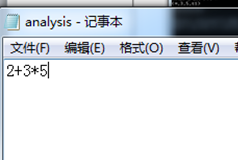词法分析结果：最终得到的结果：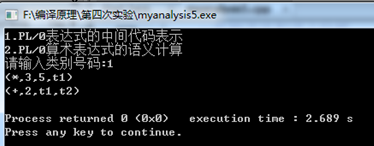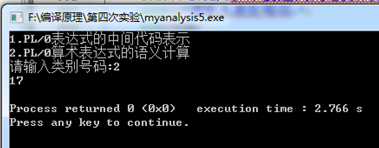待输入的文件流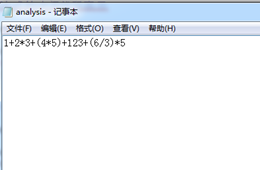词法分析结果：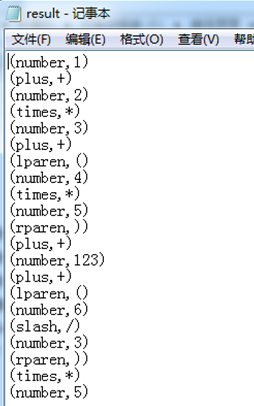最终得到的结果：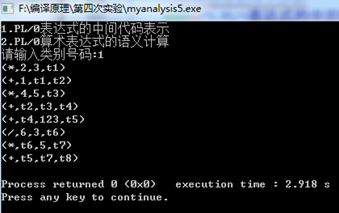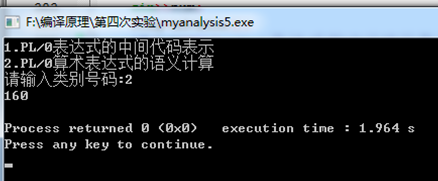展开全文• 通过设计、编制、调试一个算术表达式的语法及语义分析程序，加深对语法及语义分析原理的理解，并实现词法分析程序对单词序列的词法检查和分析。计算器
• 在实验一词法分析的基础上，以词法分析输出结果（单词串或者成为多元式序列）作为该语法语义分析器的输入，最后输出中间代码四元式序列，并计算出表达式最后的结果。（共8个上机学时，时间不够的请自己课下找时间补...
• 在实现词法、语法分析程序的基础上，编写相应的语义子程序，进行语义处理，加深对语法制导翻译原理的理解，进一步掌握将语法分析所识别的语法范畴变换为某种中间代码（四元式）的语义分析方法，并完成相关语义分析器...
• （2）阅读语义分析程序，加上你自己的理解。尤其要求对相关函数与重要变量的作用与功能进行稍微详细的描述。若能加上学习心得则更好。TINY语言请参考《编译原理及实践》第6.5节；PL/0语言请参考相关实现文档。 ...
• 语义分析 编译原理 北邮 大三 实验要求：编写语义分析程序，实现对算术表达式的类型检查和求值。要求所分析算术表达式由如下的文法产生。 实验要求：用自底向上的语法制导翻译技术实现对表达式的分析和翻译。 (1) ...
• 阅读已有编译器的经典语义分析程序，并测试语义分析器的输出。 实验内容 （1）选择一个编译器，如：TINY或PL/0，其它编译器也可（需自备源代码）。 （2）阅读语义分析程序，加上你自己的理解。尤其要求对相关...
• 用Javacc实现MiniC的词法分析、语法分析、语义分析。在词法分析部分根据单词的构词规则分类，输出<单词种别，单词自身值>二元式...语义分析部分是在语法分析的基础上根据属性文法制导翻译，进行语义分析输出四元式。
• 十、设计SAMPLE语言的语法、语义分析器，输出四元式的中间结果。 检查要求： a)启动程序后，先输出作者姓名、班级、学号（可用汉语、英语或拼音）。 b)请求输入测试程序名，键入程序名后自动开始编译。 c)输出四元式...
• 在语法树中去掉一些对翻译不必要的信息后，获得 的更有效的源程序的中间表示，这种经过变换后的语法 树称为抽象语法树。 内部结点代表操作符，它的孩子代表对应的操作数。 树表示法: 索引法: 2. 举例说明什么是DAG...
• 阅读已有编译器的经典语义分析程序，并测试语义分析器的输出。 三、实验内容 （1）选择一个编译器: 选择一个编译器，如：TINY或其它编译器也可（需自备源代码）。 我所选用的是TINY源码进行分析，目录结构如下，...
• 注：本文记录　WHUT-计算机学院-编译原理　课程　课内实践 ... 纸上得来终觉浅，觉知此事需躬行！ 0、笔者： 编译原理课程一共有两次实验和一次课内... 赋值语句的语义分析程序设计与实现 而课内实践的内容是：WHI...赋值语句
•  语义分析编译的第三步，它的主要任务是：1.收集标识符的属性信息信息，如收集种属信息（简单变量、复合变量（数组等）、过程），类型信息，存储位置，长度，值，作用域，参数，返回值等。在语义分析阶段收集的...
• //词法分析得到的单词个数 string str; //输入串 char *key[ 10 ]={ "main" , "void" , "int" , "char" , "printf" , "scanf" , "else" , "if" , "return" }; char Word[ 20 ],ch; string Vn= "AETFV" ; /...计算器
• 编译原理》课程实验报告 实验名称：语义分析 姓名： 学号： 地点： 四教302 教师： 院系： 专业： 计算机科学与技术15-1 ...
• 语法制导的定义：为每一个产生式编制一个语义子程序。当一个产生式获得匹配时，调用相应的语义子程序实现语义...#语义分析，计算器 import pandas as pd data={'id':['s5',' ',' ',' ','s5',' ','s5','s5','s5',...
• PL/0语言是Pascal语言的一个子集，我们这里分析的PL/0的编译程序包括了对PL/0语言源程序进行分析处理、编译生成类PCODE代码，并在虚拟机上解释运行生成的类PCODE代码的功能。 PL/0语言编译程序采用以语法分析为核心...
• 　这次实验的实验目的其实很明确——对源码进行语义分析输出语义分析结果，并要求有适当的错误处理机制。可是指导书上实验目的要求自己分析，我的分析结果：本次实验要求自己定义上次实验的语法分析的文法的SDD，...java Java JAVA
• 采用递归下降语法制导翻译法,对算术表达式、赋值语句进行语义分析并生成四元式序列。   实验的输入和输出 输入是语法分析提供的正确的单词串，输出为三地址指令形式的四元式序列。 例如：对于语句串 begin a:...
• 编译原理实践：C++实现语义分析器（学习笔记）实践说明输入举例（input.txt）输出举例（output.txt）编程平台代码实现基本思路语义分析部分预定义主函数定义语义分析部分函数语法分析主函数语句串分析函数语句分析...c++ 编译器
• 采用实验1的简单语言，设计并实现含多条简单赋值语句的语法语义分析程序，要求采用递归下降翻译法。 注意与实验1、2的衔接。 using System; using System.Collections.Generic; using System.IO; using System....
• 说在前面 实验四哪里去了？ : 我也不会写，所以我也是抄的 Q：实验五是我自己写的吗？ : 不是。因为我之前写的Tiny，但是到了这个实验，...这次的语义分析器也是借鉴了其完整的C-编译器的部分，进行了相应的修改。 ......